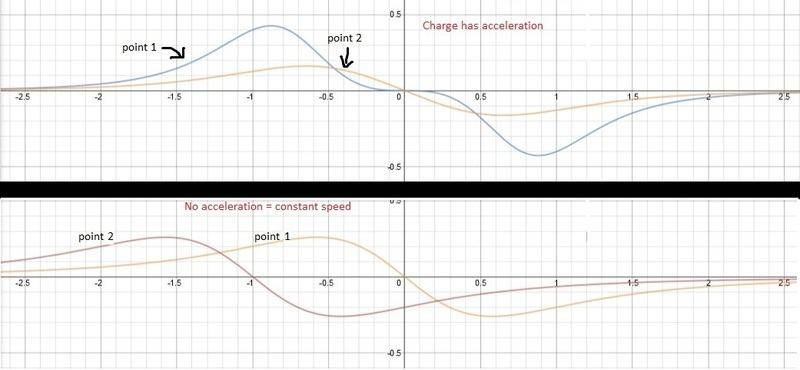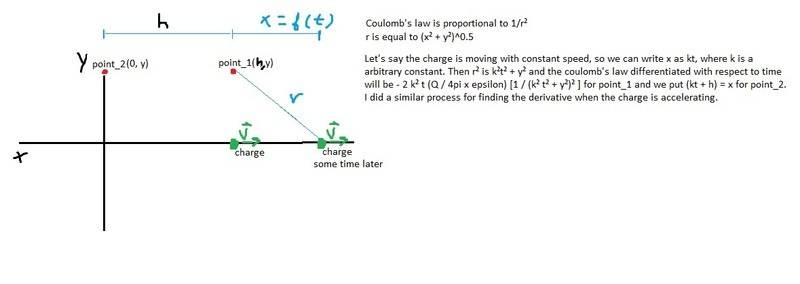# Electric field and electromagnetic waves

• kent davidge

#### kent davidge

(sorry for my poor english) I've read that a electromagnetic wave only exists in a particular point of space if in such a point there is a nonlinear change of the electric field in respect to time. Then I took a graph calculator and I derived Coulombs equation for the electric field. The results are shown below.
What surprised me was that when the charge is accelerating, the curve of the rate of change of the field in two points on the same horizontal axis has different forms (maximum points are different). Has it anything to do with the statement I mentioned above? Also, even so I don't know why would it be a wave if these two points has different rate of change at the same time.Last edited:
Start from the beginning. What kind of charge are you accelerating? (a point charge?) What is the path of the charge?
What are you plotting? What are the x and y axes on your plot, and what is point 1 and point 2?
What do you mean by nonlinear?

Last edited:
Yes. It's a point charge. By nonlinear I mean a plotted graph E x t where the function isn't a line.But why I can't use Coulomb's law if the distance is a function of time and I properly derived the field in respect to time?

Because Coulomb's law is only valid for static charges. For slow moving charges (compared to the speed of light), it can be fairly accurate, but you can't get waves from Coulomb's law. A moving charge will create a current and a magnetic field. Have a look at Maxwell's equations. If you are in self study, be patient, because normally students learn electrostatics, and then magnetostatics, before learning electrodynamics.

•davenn
Ok. What must happen with the magnitude of the electric field in those two points of my example right before and after a wave passing through them?

You only get a wave when you have an accelerating charge. The constant moving charge will have a field, but it won't be a wave.
According to special relativity, no information can travel faster than the speed of light. That means if you accelerate a charge, it will take some time (distance divided by c) before the field is affected at some distant point. Coulomb's law doesn't take this into account.
This might be useful
•